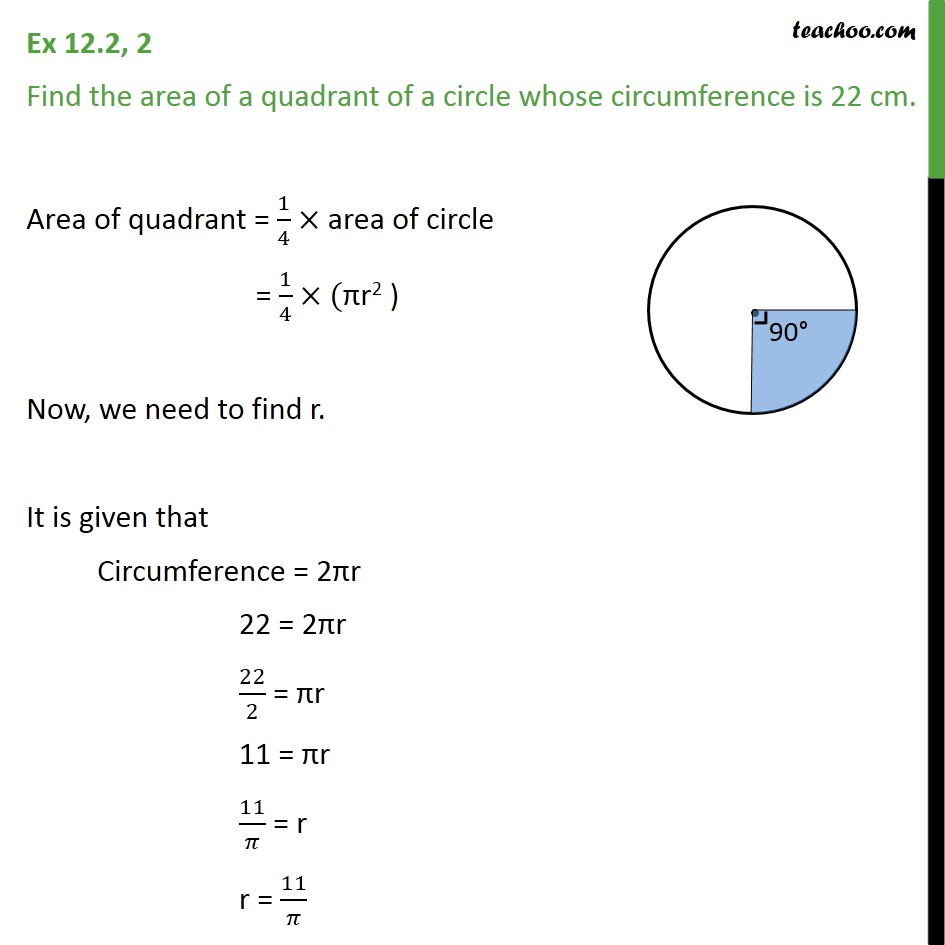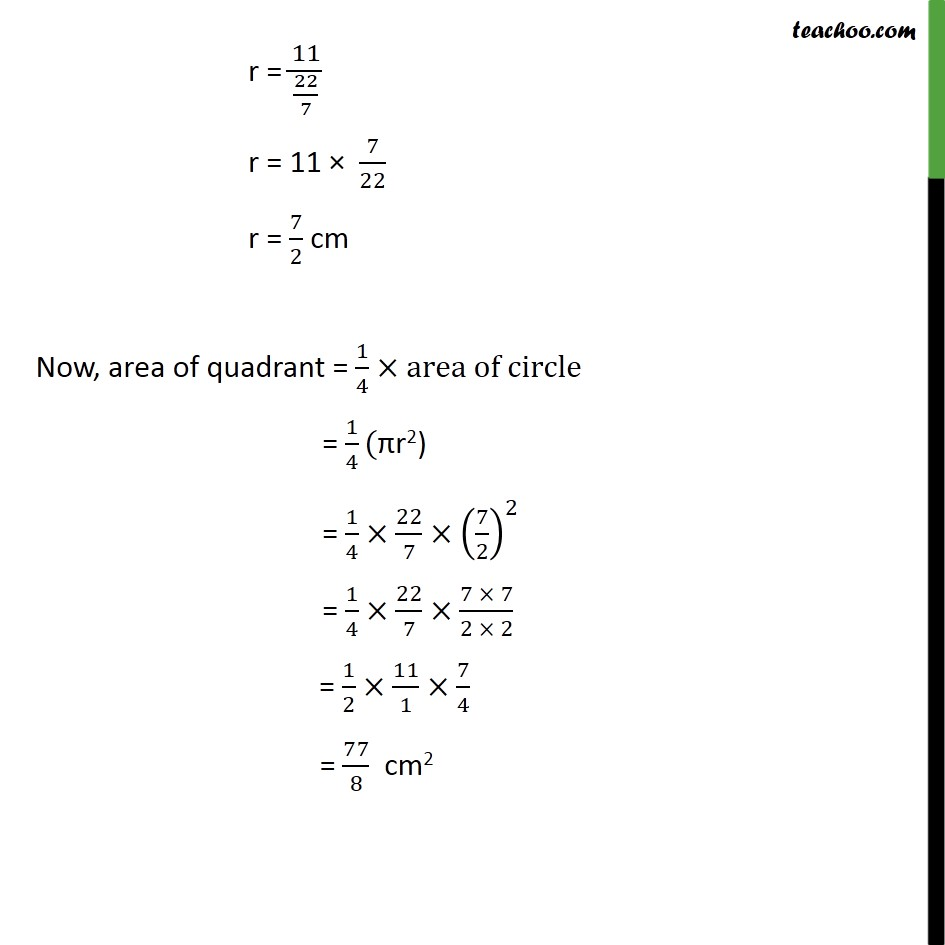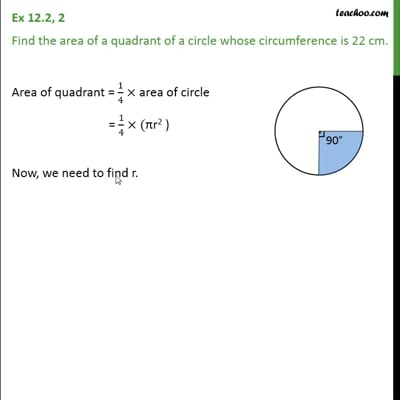Ex 12.2

Chapter 12 Class 10 Areas related to Circles
Serial order wiseThis video is only available for Teachoo black users

Introducing your new favourite teacher - Teachoo Black, at only ₹83 per month

### Transcript

Ex 12.2, 2 Find the area of a quadrant of a circle whose circumference is 22 cm. Area of quadrant = 1/4 area of circle = 1/4 (" "r2 ) Now, we need to find r. It is given that Circumference = 2 r 22 = 2 r 22/2 = r 11 = r 11/ = r r = 11/ r = 11/(22/7) r = 11 7/22 r = 7/2 cm Now, area of quadrant = 1/4 area of circle = 1/4(" "r2) = 1/4 22/7 (7/2)^2 = 1/4 22/7 (7 7)/(2 2) = 1/2 11/1 7/4 = 77/8 cm2